### Adding a terrain Blend node

To add a node, right click in the Graph Editor and select Create Node  > Terrain Composition > Blend.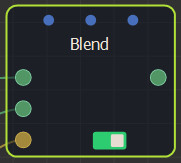Double click on the node to open its parameters: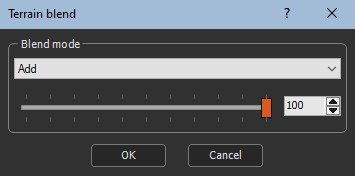### Editing a terrain Blend node

The blend mode which indicates how the second terrain is blended with the first terrain using blending coefficient from 0 to 100.

•  When the blending coefficient is 100, blending is carried out in its entirety.
•  When the blending coefficient is 0, no blending is carried out and the result is the same as the first input terrain.

When an optional mask is connected, the value of this mask at each of its points is multiplied by the selected blending coefficient to obtain the final blending coefficient. For example, if a blending coefficient of 80 is selected and the optional mask contains values equal to 0.5, the final blending coefficient of 0.5 x 80 = 40 is achieved.

• An entirely black mask results in no blending, which is the same result as the first input terrain.
• Similarly, an entirely white mask has no influence on the blending coefficient and the same result is obtained as if no mask is connected.

Select the blend mode to apply to the composition.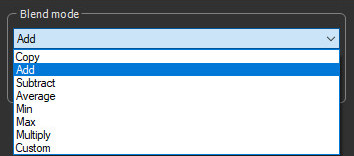Here is the result of the different blend modes, starting from these two imput terrains.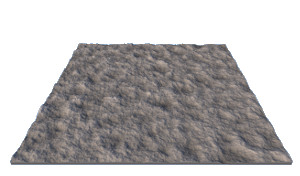Base terrainSecond terrain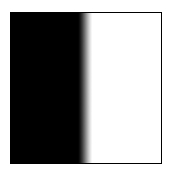Mask used to drive the blend

### Copy

Copy: The second terrain is copied in place of the first terrain. This mode is useful when a blending coefficient other than 100 is selected, or when an optional mask is connected. For example, with a blending coefficient of 25, 1/4 of the first terrain and 3/4 of the second terrain is obtained. With a blending coefficient of 50, a balanced blend of the two terrains is achieved.Add: The output terrain is the sum of the two input terrains. This is the default mode.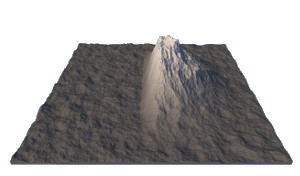### Subtract

Subtract: The output terrain is the difference between the first terrain and the second terrain.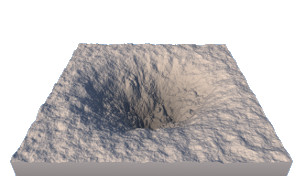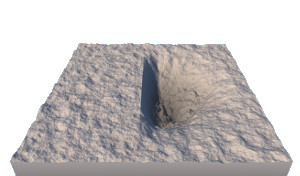### Average

Average: The output terrain is the average between the first terrain and the second terrain.

The same result is obtained as using the Copy option with a blending coefficient of 50.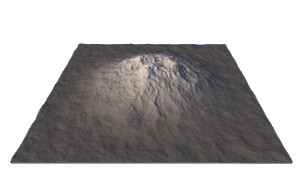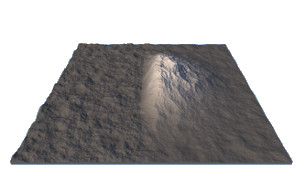### Min

Min: At each vertex, the output terrain is equal to the lowest input terrain.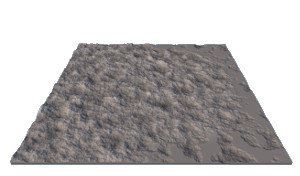### Max

Max: At each vertex, the output terrain is equal to the highest input terrain.### Multiply

Multiply: When this mode is selected, an additional Scale parameter appears.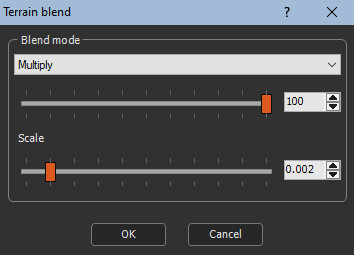At each vertex, the height of the output terrain is equal to the product of the heights of the two terrains multiplied by the Scale parameter. For example, with the first terrain is at height 1000, the second terrain is at height 800, and the Scale parameter is equal to 0.002, the height of the output terrain is equal to 1000 x 800 x 0.002 = 1600.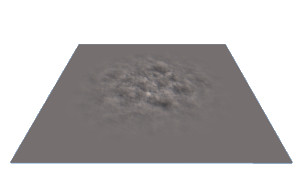### Custom

Custom: When this mode is selected, two additional parameters appear:At each point, the height of the output terrain is equal to the height of the first terrain multiplied by the Coefficient for terrain 1 parameter, plus the height of the second terrain multiplied by the Coefficient for terrain 2 parameter.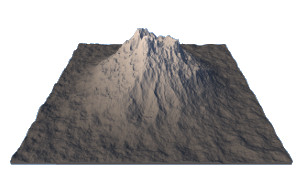#### Terrains with color maps

When both input terrains have a color map, an additional Blend color map check box appears: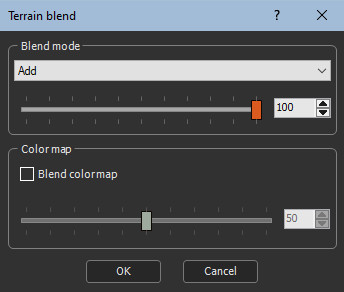• When the Blend color map check box is not checked, the output terrain retains the color map of the first input terrain.
• When the Blend color map check box is checked, the color map of the output terrain is a blend of the color maps of the input terrain. The blending coefficient is defined between 0 (only the color map of the first terrain ) and 100 (only the color map of the second terrain ).

### Parameters

Parameter Use
Copy The second terrain is copied in place of the first terrain.
Add The output terrain is the sum of the two input terrains.
Subtract The output terrain is the difference between the first terrain and the second terrain.
Average The output terrain is the average between the first terrain and the second terrain.
Min At each vertex, the output terrain is equal to the lowest input terrain.
Max At each vertex, the output terrain is equal to the highest input terrain.
Multiply At each vertex, the height of the output terrain is equal to the product of the heights of the two terrains multiplied by the Scale parameter.
Custom Blends the terrains according to custom coefficients.
Coefficient for terrain 1 The coefficient by which the height of the first input terrain is multiplied.
Coefficient for terrain 2 The coefficient by which the height of the second input terrain is multiplied.
Blend color map Blends the color maps of both input terrains.